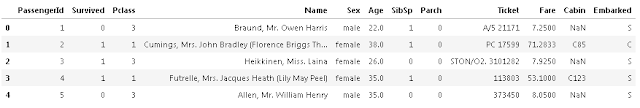# Logistic Regression :

Welcome everyone, this is first post of LOGISTIC REGRESSION. In the previous post we see Linear regression.
OK Let's start :
For this post we will  working with the Titanic Data Set. This is a very famous data sets and very often is a student first step in machine learning section.
We will  be try to predict a classifications- survival or deceasd. Let's begin our understanding of implementing Logistic Regression in Python for classifications.
We will  use a "semi-clean" version of the titanic data set, if you use the data set hosted directly on Kaggles, you may need to do some additional cleaning not shown in this lecture notebooks.

## Import Libraries

Let's import some library to get start:s
```import pandas as pd
import numpy as np
import matplotlib.pyplot as plt
import seaborn as sns
%matplotlib inline```

## The Data

Let's start by reading in the titanic_trains.csv files into a pandas dataframes.
`train = pd.read_csv('titanic_trains.csv')`
`train.head()`fig 01) Logistic Regression-plot and explained Algorithms

# Exploratory Data Analysis:

Let's begin some exploratory data analysis! We will start by checking out missing data.

## Missing Data

We can use seaborn to create a simple heatmap to see where we are missing datas .
`sns.heatmap(trains.isnull(),yticklabels=False,cbar=False,cmap='viridis')`

Roughly 30 percent of the Ages data is missing. The proportion of Ages missing is likely small enough for reasonable replacement with some form . Looking at the Cabin columns, it look like we are just missing too much of that data to do something useful with at a basic levels. We will probably drop this laters.
Let's continue on by visualizing some more of the data, Check out the graph for full explanations over these plots.

```sns.set_style('whitegrid')
sns.countplot(x='Surviveds',data=train,palette='RdBu_r')```
```sns.set_style('whitegrids')
sns.countplot(x='Surviveds',hue='Sex',data=trains,palette='RdBu_r')```
```sns.set_style('whitegrids')
sns.countplot(x='Surviveds',hue='Pclass',data=trains,palette='rainbow')```
`sns.distplot(train['Ages'].dropna(),kde=False,color='darkred',bins=40)`
`train['Ages'].hist(bins=40,color='darkred',alpha=0.8)`
`sns.countplot(x='SibSp',data=trains)`
`train['Fare'].hist(color='green',bins=40,figsize=(9,4))`
IN THE NEXT POST WE WILL SEE CUFFLINKS FOR PLOT